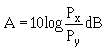frequency at which the magnitude of the fiber Frequency response has decreased to one half its zero-frequency value. "> AnswersCustom SearchANSWERS TO QUESTIONS Q1. THROUGH Q38. A1. Cladding diameter, core diameter, numerical aperture, and mode field diameter. A2.A3. Yes. A4. Launch spot size and angular distribution. A5. Low-order modes. A6. Overfilled. A7. Second-order mode. A8. Free-form loop and mandrel wrap. A9. Depressed-clad. A10. Higher. A11. The -3 decibel (dB) is the lowest frequency at which the magnitude of the fiber Frequency response has decreased to one half its zero-frequency value. A12. Differential group delay τ(λ). A13. The wavelength range of the optical source(s) used. A14. To reduce system attenuation and coupling loss resulting from poor fiber fabrication. A15. The cladding diameter is the average diameter of the cladding. A16. Multimode core-cladding concentricity error is the distance between the core and cladding centers expressed as a percentage of core diameter while the single mode core-cladding concentricity error is just the distance between the core and cladding centers. A17. The near-field region is the region close to the fiber-end face. A18. The core diameter is defined as the diameter at which the near-field intensity is 2.5 percent of the maximum intensity. A19. The far-field region is the region far from the fiber-end face. A20. Increase. A21. By disturbing the fiber's mode power distribution (MPD). A22. Reduce the stability of the system source and increase the signal noise present at the optical detector. A23. The ratio of reflected optical power to incident optical power. A24. No. A25. No. A26. When installed optical fiber cables or links are 50 meters or more in length. A27. Rayleigh scattering and Fresnel reflection. A28. Optical fiber cables, connectors, splices, mounting panels, jumper cables, and other passive components. A29. A point defect. A30. To reduce the effect of the initial reflection at the OTDR. A31. Forward optical power and backscatter capture coefficient. A32. By performing the OTDR attenuation measurements in each direction along the test fiber. A33. A point defect. A34. (a) Reflective, (b) nonreflective, and (c) nonreflective. A35. A point defect may exhibit apparent gain because the backscatter coefficient of the fiber present before the point defect is higher than that of the fiber present after. A36. An exaggerated loss. A37. When an installed optical fiber cable or cable plant is less than 50 meters in length. A38. With an OTDR unless it is less than 50 meters in length. If it is less than 50 meters in length, continuity should be verified with a flashlight.Integrated Publishing, Inc. - A (SDVOSB) Service Disabled Veteran Owned Small Business